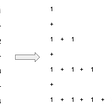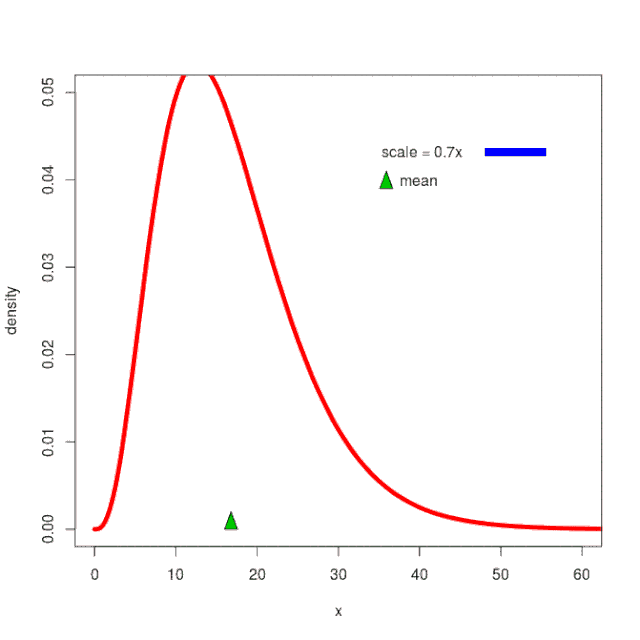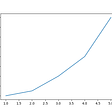# Probability theory: Probability density function

This is part of the course “Probability Theory and Statistics for Programmers”.

The probability density function is a derivative of the distribution function, which characterizes the density with which the values of the random variable are distributed at a given point.

Let’s take a look at the simple example. We have distribution function and we should find the probability of falling in the interval between a and b. This probability is simply area lying under the curve from a to b. In order to find definite integral, we will use a magic function from numerical analysis domain:)

If we have distribution function only we also can find the probability of falling into the interval.

Next part ->

Reach the next level of focus and productivity with increaser.org.

--

--

--

Indie hacker behind increaser.org. More at radzion.com

Love podcasts or audiobooks? Learn on the go with our new app.

## Probability theory: Uniform distribution## When Bayes, Ockham, and Shannon come together to define machine learning## Markov Chain Analysis and Simulation using Python## Euler Totient Function in its core!## Probability theory: Exponential distribution## Sum of first n natural numbers (How?)## Statistics: Hypothesis testing, power of the testIndie hacker behind increaser.org. More at radzion.com

## Key probability distributions and how to use them in python.## How to make interpolated lines in Python